The State of MathML : Mathematically Speaking (and Stuttering)

You are here: irt.org | Articles | Markup Languages | The State of MathML : Mathematically Speaking (and Stuttering) [ previous next ]

Published on: Sunday 19th March 2000 By: Pankaj Kamthan

Introduction

Since its inception in the early 1990's, the Web has become a widely accepted medium for archiving, disseminating and communicating information. In the last few years, the Web technology is moving into the arena where it can be directly useful as a tool for educational, research and commercial purposes. The development of the MathML is a major step in the direction of providing a means of [re]presenting mathematical notation on the Web.

This work examines the state of MathML, particularly the issues regarding its deployment, in the light of other technologies. The purpose of this exercise is to objectively assess the current situation with the goal to increase public awareness, rather than a marketing-oriented critique. We assume the reader has basic background in XML syntax and MathML. An elementary knowledge of some other XML vocabularies will also be useful, but is not required. For a tutorial introduction to MathML, see A Gentle Introduction to MathML.

A Brief History of MathML

From the very beginning, [re]presenting Mathematics on the Web proved to be a nontrivial task. In 1993, ideas for embedding Mathematics in HTML emerged in the HTML+ and subsequently in HTML 3.0 in 1994. Later, in 1996, formal support was added in HTML 3.2. However, due to lack of interest from major browser vendors, it failed to get wide acceptance.

In 1997, a Math Working Group was constituted at the World Wide Web Consortium (W3C). A solution based on Standard Generalized Markup Language (SGML) was felt to be too complicated, and it was decided that a mathematical markup language (under the name MathML) for describing mathematical content be based on XML syntax. In April 1998, MathML 1.0 Specification was announced as a W3C Recommendation, and in July 1999, it was revised to become MathML 1.01 Specification. Currently, work on MathML 2.0 is under development.

A MathML Tour

Presenting mathematics is one problem. Another problem is to search through such mathematical expressions or cut-and-paste them into computational software for further manipulation. Therefore, the markup needed to be both visually robust and meaningful. This led to the introduction of two types of markups in MathML: Presentation Markup and Content Markup.

Presentation Markup captures the notational structure of an expression. As a general rule, each presentation element corresponds to a single kind of notational "schema" such as a row, a superscript, and so on. Content Markup captures the mathematical structure of an expression. The majority of content elements correspond to a wide variety of operators, relations and named functions from topics typically found at the high school level mathematics, such as addition, square root, and so on.

MathML Issues

Several issues have plagued widespread acceptance of MathML. They range from technical (authoring, serving, rendering), to social (public image), and to political (support). In the foregoing sections, we examine these in detail.

MathML Authoring

One comes across assertions, such as, MathML is "a simple way ... to include Math[ematics] in Web pages by hand" contrary to "it is anticipated that, in all but the simplest cases, authors will use equation editors ... to generate MathML." MathML syntax, except the most trivial cases, is verbose, and therefore naturally lends itself for machine-processing as shown in the next example.

EXAMPLE 1. The following represents Presentation and Content Markup, respectively, of the well-known Quadratic formula:Presentation Markup (MathML source)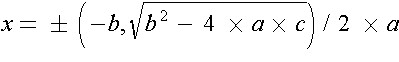Content Markup (MathML source)

Mathematics-Text Synergy in MathML Authoring

An authoring process may involve the following steps:

1. Create the document (with no mathematical content) using some word processor.
2. Create mathematical objects separately using an authoring software (popularly known as "equation editors").
3. "Embed" the results of Step 2 in Step 1 at appropriate places.

This works well but only in the simple cases where one comes across a few mathematical objects in an entire document. As an example, one can use Microsoft Word for Step 1 and MathType for Step 2. However, this does not scale well with documents that contain complex mathematics, such as, theorems that mix text with mathematical expressions naturally (for an example, see here).

The approach, therefore, is not to treat mathematical objects separately in an isolated fashion in the typographic process, but to include mathematical notation as one "moves along" when typing. This style of authoring is common with the users of LATEX, where an authoring "flow" is maintained by using the mathematical "delimiters", such as, \$...\$ for inline and \[...\] for display objects. This flexibility is yet to be achieved with MathML, though some software such as the WebEQ Wizard do provide a partial solution.

MathML [Ab]use

The policy "let us represent everything in MathML" just because "MathML is there" is more abuse than proper use. Use of MathML Presentation Markup for simple mathematical objects is an "overkill," since MathML markup is verbose compared to ASCII.

EXAMPLE 2. Consider the expression 1 + sin(x). The following is the corresponding MathML Presentation Markup for it:

<?xml version="1.0"?>
[itex]
<mrow>
<mi>1</mi><mo>+</mo><mi>sin</mi><mo>&ApplyFunction;</mo><mo>(</mo><mi>x</mi><mo>)</mo>
</mrow>
[/itex]

Use of the operator element <mo> for the addition operation, and identifier <mi> element for the Sine function, provides only "vague" semantics. Presentation Markup is not very useful for search engines (where it is assumed that they are searching element names and not what they encapsulate). However, the use of MathML Content Markup may be justified, as it associates a lot more semantics, by its very intent:

<?xml version="1.0"?>
[itex]
<apply><plus/><cn>1</cn><apply><sin/><ci>x</ci></apply></apply>
[/itex]

MathML Software : No Free Lunch

Outside the realm of W3C, almost all MathML software available is commercial. These commercial efforts also seem to be disparate, existing as independent islands of initiatives, lacking a combined major effort needed for one of the largest XML vocabularies to date. There is lack of Open Source software for authoring or translating or rendering, for MathML in general, and for MathML Content Markup, in particular. For those that are available, authoring process is made tedious by some solutions which enforce their own syntax (WebEQ and EzMath), which is translated to MathML but is incomplete. Furthermore, the rendering quality in some cases (compared to printing) is unacceptable.

There is no free lunch, and one should not be expected. But freely available and Open Source software has been one of the primary reasons for a broad utilization and success of HTML as a Web data format. The same factor is crucial for widespread adoption of XML, and in particular MathML, as well.

Mathematical Markup and Semantics

Elements of MathML Content Markup, which cover the basic mathematics, is too constrained for higher-level mathematics. For example, there is no MathML Content element to explicitly represent the "rank of a matrix operator" of Linear Algebra. MathML allows the list of content elements to be extended (in our case, to include a "rank of a matrix operator element") using the <annotation-xml> element with the OpenMath encoding Content Dictionary (in our case, BasicLinAlg) in conjunction with the <semantics> element. However, there are cases, such as the widely-used Frobenius-Perron operator of Ergodic Theory, where such a Content Dictionary may not exist in a specific encoding.

Without sufficient support for Content Markup, mathematical "meaning" in corresponding markup diminishes. This could be somewhat improved by appropriate use of a metadata mechanism, such as Resource Description Framework (RDF). RDF is a foundation for processing metadata. It provides interoperability between applications that exchange machine-understandable information on the Web. The RDF Syntax, based on XML, expresses and transports metadata in a manner that maximizes the interoperability of independently developed Web servers and clients. RDF Schemas define a collection of information about classes of RDF nodes, including properties and relations. RDF schemas are specified using a declarative representation language influenced by ideas from knowledge representation such as semantic nets, frames, and predicate logic, as well as database schema representation models such as binary relational models, and graph data models. A widely-used example is the Dublin Core (DC) schema.

MathML-specific metadata has not been yet formalized. However, the next example shows the utility of such an endeavour.

EXAMPLE 3. This example embeds both MathML Content Markup, and RDF markup (in serialization syntax) using the Dublin Core metadata, of the Quadratic Formula in an Extensible HyperText Markup Language (XHTML) 1.0 document:

<?xml version="1.0" encoding="UTF-8" ?>
<!DOCTYPE html PUBLIC "-//W3C//DTD XHTML 1.0 Strict//EN"
"http://www.w3.org/TR/xhtml1/DTD/xhtml1-strict.dtd">

<html xmlns="http://www.w3.org/1999/xhtml" xml:lang="en" lang="en">
<body>

<!-- RDF Description of the Quadratic Formula. -->
<rdf:RDF
xmlns:rdf="http://www.w3.org/1999/02/22-rdf-syntax-ns#"
<!-- Quadratic Formula RDF/DC Markup (within, and including the <rdf:Description> tag) here. -->
</rdf:RDF>

<!-- MathML Content Markup of the Quadratic Formula. -->
<math xmlns="http://www.w3.org/1998/Math/MathML">
<!-- Quadratic Formula Content Markup (within, and including the <reln> tag) here. -->
[/itex]

</body>
</html>

Legacy Documents' Conundrum

Translating the existing base of (a very large collection of) legacy documents to MathML is an important issue. Such documents are primarily in TEX (or one of its family members, such as LATEX) and Microsoft Word.

There are several questions that need to be asked (and answered to a satisfactory extent):

• Timeliness/Stability. Is the time ripe for legacy document conversion to MathML? See the section on Image is Everything. Will this result in a "standard hopping" (from * to MathML to yet something else)? This can be a major waste of resources. It is a "give and take" situation, with no guarantees for success.
• Competition. "Why not just convert the documents to Portable Document Format (PDF)?" By using PDF one loses the advantages that XML offers, but there are other benefits. For example, with PDF the production cycle is straightforward, and there are almost no rendering uncertainities at the delivery-end. It thus becomes a question of priorities.
• Procedure. Manual translation is possible but hardly practical. Therefore, *-to-MathML-type convertors are being developed. XSL Transformations (XSLT) can also be used to translate XML documents in other vocabularies to MathML. A large-scale translation, even if automated, can be a mammoth effort, and this should be taken into consideration. Accuracy is another issue. Such translations suffer from the limitation that they can could only be translated to the Presentation Markup, thus losing the advantage that the Content Markup offers.

MathML Rendering Fiasco

Even after an year and a half in existence as a W3C Recommendation, and multiple revisions thereafter, rendering support for MathML in widely-used browsers is practically nonexistent. Among freely available browsers, only the W3C test-bed browser Amaya supports almost all of the Presentation Markup. This is reminiscent of the introduction of mathematical notation in HTML 3.0 (now deprecated) which was supported only in the W3C test-bed browser Arena. The situation is improving with the MathML in Mozilla effort, but implementations are premature and the release date of Mozilla itself is unclear. Also, the current support is only for Presentation Markup.

There are browser plug-ins available, such as, IBM techexplorer Hypermedia Browser with a large support for both MathML Content and Presentation Markup. However, plug-in use suffers from historical problems such as making the browser monolithic and being a performance hog. Furthermore, it is extremely impractical approach for documents containing several mathematical objects (for an example, see here).

MathML Training (The Lack Thereof)

With MathML, we now have a mechanism of putting mathematical objects on the Web. However, there is an apparent lack of training that would lead to the realization that having mathematical notation on the Web marked-up in MathML would indeed be radically different, and useful. This situation is likely to improve as MathML enters the "consumption phase," finding its place, for example, in books and classrooms.

Image is Everything

A widespread acceptance is necessary for any technology's widespread use. Acceptance may lead to adoption, which in turn depends strongly on public perception. Some public "misconceptions" are discussed below in detail:

• MathML is Experimental (The "Wait-and-See" Mode). There is some truth to this impression depending on what aspect we look into. MathML, as a language, has seen a few stages since it was first announced as a W3C Recommendation and has considerably matured in this period of evolution. However, MathML is a low-level markup and therefore requires application software to be useful. MathML development, as this article points out, is in an unsatisfactory state. For a developer, the cost-result-success (for example, what is the cost, how quickly can the results be obtained, and how well can they be presented) trio is important. Due to the lack of freely available software for authoring, and insufficient support for rendering, convincing arguments against this perception become all the more weak.
• MathML is (just) "TEX for the Web." TEX is very widely used system for encoding mathematics. However, it encodes only the presentation of mathematics, and does not encode either semantic or structural information. There are cases where needs (such as making use of semantic or structural information) arise that require a better solution. For example, a computer algebra system is not primarily interested in how a mathematical expression is displayed. MathML attempts to provide a presentation mechanism that not only has the expressive capabilities of TEX, but also has enough information in it so that the presentation is accessible to the visually impaired, and capable of doing a good job of linebreaking expressions, since the author can not know in advance the window size and font size in which the MathML should render. In addition to a presentation interface, it is necessary to provide mechanisms for encoding mathematical semantic content, and controlling the interface between the embedded MathML fragment and the browser processing the containing page. Therefore, MathML is a low-level markup and should be viewed as roughly comparable to PostScript, and not as a competitor of TEX.

As indicated in this work, there are some genuine concerns, which go beyond just misconceptions. The purpose of discussing these is to provide an objective view, rather than inflate a negative public perception, and therefore should be seen as such.

The Nature of Mathematics

[Re]presenting mathematical notation is only part of the larger picture. This picture includes other aspects of mathematics which these notations are supposed to represent. This section discusses a few of these in detail.

"XMLize" and Visualize

Geometry plays a significant role in various areas of mathematics. Geometrical information comes into play in various ways, including the following:

• Schematics. Drawing that represents physical objects, such as a circle, a M�ebius strip, a torus.
• Graphs. Graph of a real polynomial.
• Geometrical Representation of Computational Data. Simulation plots, statistical data plots, such as, a pie chart or a histogram.

Support for geometry in MathML is limited. In simple cases, such as basic commutative diagrams or other algebraic relationships, MathML could be used. Other cases, such as graphs, or geometric shapes, are beyond the scope of MathML and can not be represented. Therefore, by necessity, one has to resort to other techniques.

The issue of representing 2D geometrical structures on the Web may somewhat be resolved with the ascent of Scalable Vector Graphics (SVG). SVG is a language for "describing two-dimensional vector and mixed vector/raster graphics in XML." The next example illustrates the possibilities.

EXAMPLE 4. One simple and one complex geometrical structure represented in SVG.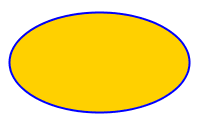Figure 1. An Ellipse (SVG source).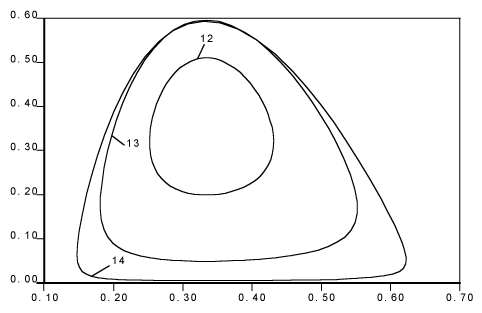Figure 2. A Phase Plot of a system of two nonlinear ODEs representing a Predator-Prey System (SVG source; 4K, Zip).

For representing 3D geometrical structures on the Web, a different vocabulary is desirable. An important advancement in this area has been Virtual Reality Modeling Language (VRML). Inspite  of the media hype, lack of native support in widely-used browsers, proprietary declarative syntax, and large file sizes, might have been some of the reasons for its limited use by general public. In the mathematical community, direct VRML authoring complex structures may be seen as inadequate (there is only so much one can do with cubes, cones and spheres). Therefore, use of mathematical software to generate these structures and subsequent translation to VRML, becomes necessary.

EXAMPLE 5.The graph of the expression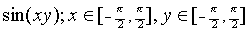... (1)

is three-dimensional in nature and can be represented in VRML (see Figure 3). MathML source for expression (1) is available.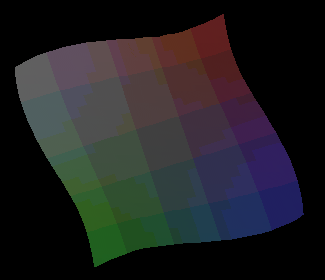Figure 3. A Sine function in 3D (VRML Source; 30K, Zip).

An "XMLization" of VRML has been initiated recently under the X3D project with the goal of designing and implementing an extensible 3D graphics specification by expressing the geometry and behaviour capabilities of VRML using XML and ensuring interoperability with other standards such as XHTML, SVG and SMIL.

Mathematics is "Living"

There are various mathematical phenomena that are dynamic in nature and need to be communicated in the form of an animation or video for a proper understanding. For example, weather data could be plotted and animated (using SMIL Animation) in SVG to show variations. Any mathematical equations involved could be represented in MathML. Such situations are common rather than an exception. The next section provides a flavour.

A Demontration of Chaos in SMIL

Iteration is an act of repeating a procedure on an object. We come across iterations everywhere. Hammering a nail, axing a log of wood, punching a number in a calculator and pressing the sin(x) button repeatedly, are all forms of iterations.

Let X be a space and f be a transformation. Then, (X,f) constitutes a dynamical system and the set {x, f(x), f2(x), ...,fn(x), where n is a nonnegative integer and x is in X} is known as a trajectory. Symbolically, we can represent the process of iteration as (a difference equation):

 xn+1 = f(xn) ... (2)

where n=0,1,2,... can be considered as a discrete "time" variable. One of the simplest dynamical systems with complex behaviour of trajectories is ([0,1],f), when f is a one dimensional nonlinear transformation such as the Quadratic map

 f(x) = a x (1 - x) ... (3)

where the parameter a is in the interval [0,4]. The iteration of f can be shown graphically. The value chosen corresponding to the figures are the following: a=1.0, a=2.0, a=2.5, a=3.1, a=3.5, a=3.57, a=3.7, a=3.9, and a=4.0. The initial point in each case is x=0.35. This represents the following phenomena:

• Fixed point. For a=2.5, iterations approach a fixed point at around x=0.6.
• Period 2. For a=3.1, iterations approach close to the fixed point value at around x=0.7, but rather than converging to this value, the trajectory moves away, and eventually converges to period two trajectory.
• Period 4 and Higher. For a=3.5, iterations result in a period 4 trajectory. As a is slightly increased further (note that the consecutive differences between the values of a are becoming smaller), higher and higher period trajectories are found until at about a=3.57 a complex trajectory with no apparent repetition (periodicity) is obtained. This is the onset of chaos (see Figure 4), which becomes more apparent with a=3.9 and a=4.0.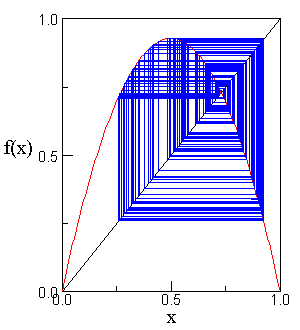Figure 4. Quadratic Map with a=3.57, x=0.35.

Here is the demo in SMIL: Quadratic Map : Transitions from a Fixed Point to Chaos (127K, Zip). It uses media components such as RealText, RealPix and RealAudio from RealNetworks. You will therefore need RealPlayer to play the presentation.

Remarks

• Equations (2) and (3) can be presented using MathML markup quite easily (but was not carried out intentionally; see the section MathML [Ab]use).
• All figures in the demo are in the GIF format which makes them larger than their PNG counterparts. This was out of necessity rather than choice. It would be encouraging to see the support for applications having "pure" marked-up text, such as, all content in MathML, SVG and SMIL, embedded in an XHTML document, with assertions based on RDF to add semantics to it.

I Talk, You Listen

It's about Mathematical Communication ... The Rest is Technology.

A concept or a phenomenon often requires more than one technology for its representation. A "convergence" of technologies to provide this "completeness" is therefore necessary. A significant development that facilitiates this interplay is XML Namespaces.

XML Namespaces provides a method for elements (and attributes) from different XML vocabularies to coexist coherently in the same document without any potential conflict. As the examples above have illustrated, use of MathML in conjunction with other XML vocabularies is important, and even necessary. Whether sufficient rendering support for a combination thereof is available is an entirely different matter. It poses a major challenge for the future, as the current situation is less than optimistic, perhaps more for political/commercial than for technical reasons.

Conclusion

MathML is a well-deserved tribute to Mathematics, the "Queen of the Sciences." However, the crowning ceremony is yet to take place. Convergence and collaboration of MathML with other technologies will be the key for a significant mathematical presence on the Web. According to the MathML 1.01 Specification, "the goal of MathML is to enable mathematics to be served, received, and processed on the Web, just as HTML has enabled this functionality for text." This goal is yet to be realized.

Acknowledgements

The RealAudio file used in the SMIL demo is by Augusto Areal. It contains a music theme composed by Giorgio Moroder for the movie Metropolis. All rights reserved. MathML authoring software MathType and WebEQ were used to generate the mathematical expressions. I would like to thank Hsueh-Ieng Pai and Martin Webb for making various editorial suggestions.

Related items

XML Namespaces : Universal Identification in XML Markup

The Emperor has New Clothes : HTML Recast as an XML Application

SVG Brings Fast Vector Graphics to Web

Time Changes Everything

P3P - What's in it for us?

XSL - What's in it for us?

RDF - What's in it for us?

MathML - What's in it for us?